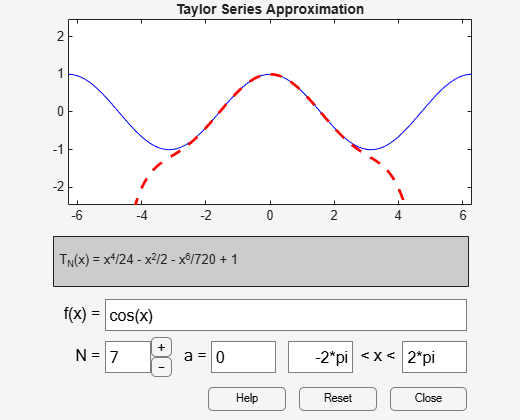taylortool

Taylor series calculator

Description

example

taylortool initiates a GUI that computes the Taylor series expansion. The GUI that graphs a function against the Nth partial sum of its Taylor series about a base point x = a. The default function, value of N, base point, and interval of computation for taylortool are f = x*cos(x), N = 7, a = 0, and [-2*pi,2*pi], respectively.

example

taylortool(f) initiates the GUI that computes the Taylor series expansion of the given expression f.

Examples

collapse all

Open a GUI that computes the Taylor series expansion. By default, the GUI shows the Taylor series expansion of the function f(x) = x*cos(x). The blue solid line shows the function f(x) and the red dashed line shows its Taylor approximation.

taylortoolNext, find the Taylor series expansion of the function cos(x).

syms x
taylortool(cos(x))Input Arguments

collapse all

Input to approximate, specified as a symbolic expression or function.